Profile MEX Functions by Using MATLAB Profiler

You can profile execution times for MEX functions generated by MATLAB® Coder™ by using the MATLAB Profiler. The profile for the generated code shows the number of calls and the time spent for each line of the corresponding MATLAB function. Use the Profiler to identify the lines of MATLAB code that produce generated code that take the most time. This information can help you identify and correct performance issues early in the development cycle. For more information on the MATLAB Profiler, see profile and Profile Your Code to Improve Performance.

The graphical interface to the Profiler is not supported in MATLAB Online™.

MEX Profile Generation

You can use the MATLAB Profiler with a generated MEX function. Alternatively, if you have a test file that calls your MATLAB function, you can generate the MEX function and profile it in one step. You can perform these operations at the command line or in the MATLAB Coder app.

To use the Profiler with a generated MEX function:

1. Enable MEX profiling by setting the configuration object property EnableMexProfiling to true.

Alternatively, you can use codegen with the -profile option.

The equivalent setting in the MATLAB Coder app is Enable execution profiling in the Generate step.

2. Generate the MEX file MyFunction_mex.

3. Run the MATLAB Profiler and view the Profile Summary Report, which opens in a separate window.

profile on;
MyFunction_mex;
profile viewer;

Make sure that you have not changed or moved the original MATLAB file MyFunction.m. Otherwise, the Profiler does not consider MyFunction_mex for profiling.

If you have a test file MyFunctionTest.m that calls your MATLAB function, you can:

• Generate the MEX function and profile it in one step by using codegen with the -test and the -profile options. If you turned on the MATLAB Profiler before, turn it off before you use these two options together.

codegen MyFunction -test MyFunctionTest -profile
• Profile the MEX function by selecting Enable execution profiling in the Verify step of the app. If you turned on the MATLAB Profiler before, turn it off before you perform this action.

Example

You use the Profiler to identify the functions or the lines of the MATLAB code that produce generated code that take the most time. Following is an example of a MATLAB function that converts the representation of its input matrices A and B from row-major to column-major layout in one of its lines. Such a conversion has a long execution time for large matrices. Avoiding the conversion by modifying that particular line makes the function more efficient.

Consider the MATLAB function:

function [y] = MyFunction(A,B) %#codegen

% Generated code uses row-major representation of matrices A and B
coder.rowMajor;
length = size(A,1);

% Summing absolute values of all elements of A and B by traversing over the
% matrices row by row
sum_abs = 0;
for row = 1:length
for col = 1:length
sum_abs = sum_abs + abs(A(row,col)) + abs(B(row,col));
end
end

% Calling external C function 'foo.c' that returns the sum of all elements
% of A and B
sum = 0;
sum = coder.ceval('foo',coder.ref(A),coder.ref(B),length);

% Returning the difference of sum_abs and sum
y = sum_abs - sum;
end

The generated code for this function uses a row-major representation of the square matrices A and B. The code first computes sum_abs (the sum of absolute values of all elements of A and B) by traversing over the matrices row by row. This algorithm is optimized for matrices that are represented in a row-major layout. The code then uses coder.ceval to call the external C function foo.c:

#include <stdio.h>
#include <stdlib.h>
#include "foo.h"

double foo(double *A, double *B, double length)
{
int i,j,s;
double sum = 0;
s = (int)length;

/*Summing all the elements of A and B*/
for(i=0;i<s*s;i++)
{
sum += A[i] + B[i];
}
return(sum);
}

The corresponding C header file foo.h is:

#include "rtwtypes.h"

double foo(double *A, double *B, double length);

foo.c returns the variable sum, which is the sum of all elements of A and B. The performance of the function foo.c is independent of whether the matrices A and B are represented in row-major or column-major layouts. MyFunction returns the difference of sum_abs and sum.

You can measure the performance of MyFunction for large input matrices A and B, and then optimize it further:

1. Enable MEX profiling and generate MEX code for MyFunction. Run MyFunction_mex for two large random matrices A and B. View the Profile Summary Report.

A = rand(20000);
B = rand(20000);

codegen MyFunction -args {A,B} foo.c foo.h -profile

profile on;
MyFunction_mex(A,B);
profile viewer;

A separate window opens showing the Profile Summary Report.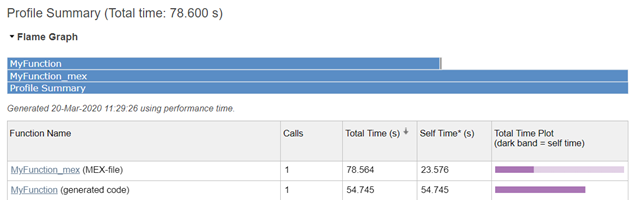The Profile Summary Report shows the total time and the self time for the MEX file and its child, which is the generated code for the original MATLAB function.

2. Under Function Name, click the first link to view the Profile Detail Report for the generated code for MyFunction. You can see the lines where the most time was spent: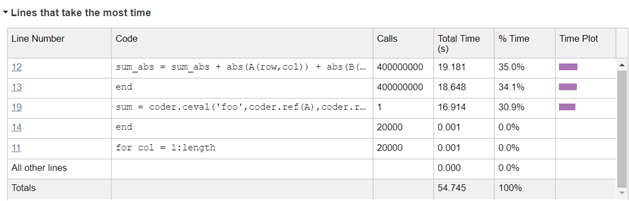3. The line calling coder.ceval takes a lot of time (16.914 s). This line has considerable execution time because coder.ceval converts the representation of the matrices A and B from row-major layout to column-major layout before passing them to the external C function. You can avoid this conversion by using an additional argument -layout:rowMajor in coder.ceval:

sum = coder.ceval('-layout:rowMajor','foo',coder.ref(A),coder.ref(B),length);
4. Generate the MEX function and profile again using the modified MyFunction.

A = rand(20000);
B = rand(20000);

codegen MyFunction -args {A,B} foo.c foo.h -profile

profile on;
MyFunction_mex(A,B);
profile viewer;
The Profile Detail Report for MyFunction shows that the line calling coder.ceval now takes only 0.653 s: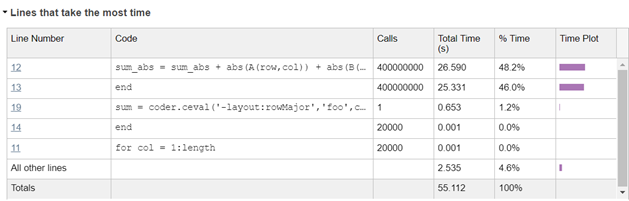Effect of Folding Expressions on MEX Code Coverage

When you use coder.const to fold expressions into constants, it causes a difference in the code coverage between the MATLAB function and the MEX function. For example, consider the function:

function y = MyFoldFunction %#codegen
a = 1;
b = 2;
c = a + b;
y = 5 + coder.const(c);
end

Profiling the MATLAB function MyFoldFunction shows this code coverage in the Profile Detail Report: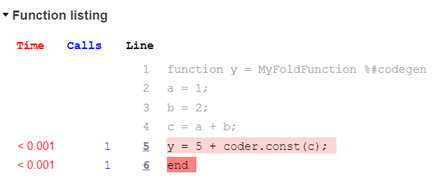However, profiling the MEX function MyFoldFunction_mex shows a different code coverage: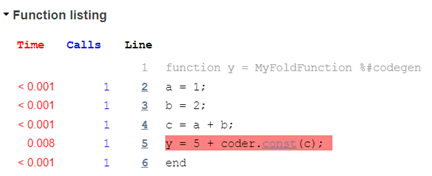Lines 2, 3, and 4 are not executed in the generated code because you have folded the expression c = a + b into a constant for code generation.

This example uses user-defined expression folding. The code generator sometimes automatically folds certain expressions to optimize the performance of the generated code. Such optimizations also cause the coverage of the MEX function to be different from the MATLAB function.